Science Journal of Physics

January 2014, Volume 2014, ISSN:2276-6367

### About the Discussion on Rewinding Timend Order

Author: Roland Meissner

Accepted 29 November, 2013; Available Online 2 January 2014

doi: 10.7237/sjp/234

#### Abstract:

The discussion about the rewinding time was initiated by Gödel in 1949. He illustrated Einstein’s equations in cylindrical coordinates and postulated a circular world line, where beginning and end are identical resulting in the fact that time can have two directions. It was not taken into account that the world line can also be spirally twined around the infinite cylinder what would mean that there is only one direction of time. There are a number of literature sources on the issue of „negative or rewinding time“. Substitutionary for the others, I would like to mention the current source in “Bild der Wissenschaft” (bdw) 04/2005 with the overview article “Die 7 Rätsel der Physik” . Aim of this essay is to prove by the example of the special theory of relativity (SRT) that there can basically not be a negative world (anti world), although the characteristic radical term for this theory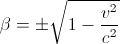(Equation: 1)

does basically have a negative algebraic sign.

2 Theorem

The following theorem is formulated:

According to the SRT, a negative area and speed does only exist, if v > c.

3 Proof

According to SRT it applies for the length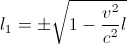(Equation: 2)

And for the time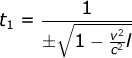(Equation: 3)

For the area, you then get: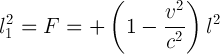(Equation: 4)

And for the speed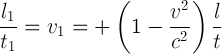(Equation: 5)

You get a negative area and speed, as easily to be seen, only and only if v > c. (2) changes into: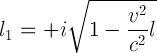(Equation: 6)

and (3) into: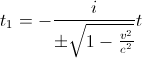(Equation: 7)

With

i-1 = -i
(Equation: 8)

(i = +√-1 imaginary unit)

While the length remains positive, the time turns into negative, but both are imaginary.

4 Discussion

At this point, we are faced with the axiomatic mathematical laws.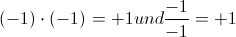(Equation: 9)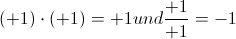(Equation: 9)

which is obviously wrong. From this, it follows that only the positive algebraic signs of the SRT are valid for a real world. Therefore, the world as a whole can not be continuously gimbally symmetric. Thus, a negative, respectively rewinding time does not exist in the real world. The double negative does not only play an important role in linguistic, but as well in philosophy (see  and ). Especially in the field of particle physics, some violations of gimbal physics became apparent. Concerning the symmetry’s violation during the neutron’s decay within the atomic nucleus (weak interaction) it was made possible to trace it back to a higher symmetry, the spheric-hyperbolic symmetry (see ). This is where cyclic and hyperbolic functions are reconciled according to Hegel’s dialectical logic . The other symmetry violations will probably be due to the fact that the world as a whole, as proven above, is not gimbally symmetric. If you exclude time from the main context and furnish it randomly, meaning unlawfully with a negative algebraic sign, negative has to be translated as wrong, abnormal, erroneous and so on. The discussion about negative time has thus to be compared to the discussion about the Aether in the 19th century.

5 References

1. Klein, F., Spiegelungen am Kreis, (Standort: Deutsche Nationalbibliothek, Leipzig)
2. Meissner, R., Grundsätzliches zur Begrenzung der Beschleunigung, zur Symmetrie und zur rückläufigen Zeit, Aachen, Shaker Verlag, 2006 Download: www.shaker.de/katalog
3. Meissner, R., Die Weltformel. Ein Formulierungsvorschlag, Tönning, Der Andere Verlag, 2010 (Deutsch und Englisch)